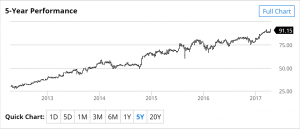24
Apr 17

## Nonstationary processes 1Nonstationary processes: as usual, to understand their role, one needs to see a couple of examples.

A stochastic process is a set of random variables indexed by time. Recall the definition of a stationary process: a) the means are constant (do not depend on time), b) the variances are also constant, and c) the covariances depend only on the distance in time between two moments. If any of these three conditions is violated, the process is called nonstationary.

Example 1. Model with a time trend. Consider

(1)$X_t=a+bt+u_t$

where$u_t$ is white noise:

(2)$Eu_t=0$$Eu_t^2=\sigma^2$ for all$t$ and$Eu_tu_s=0$ for all$t\ne s$

(these are conditions usually imposed on the error term in simple regression). (1) is nothing but a simple regression with time as the regressor. From (1) and (2) the mean of the process is

(3)$EX_t=a+bt$,

which is a straight line. As long as the slope$b$ is not zero, the means (3) vary with time, and we have a nonstationary process. We don't even have to look at other parts of the definition of a stationary process.

The behavior of the stock price of Visa, Inc. (since 2011) is a good visualization of this type of a process:Figure 1. Visa. Source: https://www.barchart.com/stocks/quotes/V